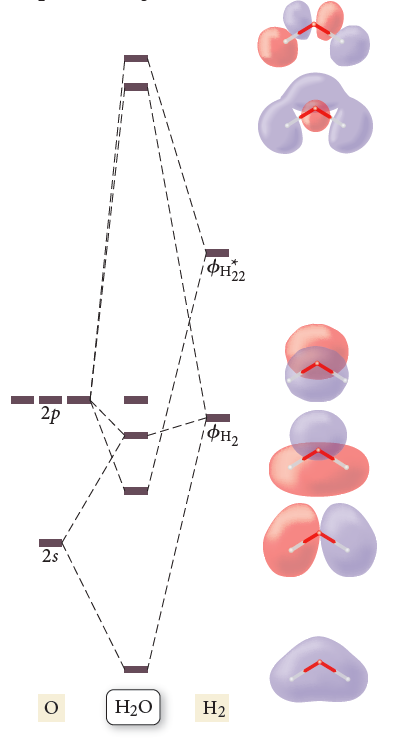⚠️Our tutors found the solution shown to be helpful for the problem you're searching for. We don't have the exact solution yet.

# Solution: The results of a molecular orbital calculation for H2O are shown here. Examine each of the orbitals and classify them as bonding, antibonding, or nonbonding. Assign the correct number of electrons to the energy diagram. According to this energy diagram, is H2O stable? Explain.

###### Problem

The results of a molecular orbital calculation for H2O are shown here. Examine each of the orbitals and classify them as bonding, antibonding, or nonbonding. Assign the correct number of electrons to the energy diagram. According to this energy diagram, is H2O stable? Explain.